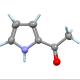Suppose we have sets$A$ and$B$ and a function$f : A \to B$ (that is,$f$’s domain is$A$ and its codomain is$B$). Suppose there is another function$g : B \to A$ such that$g(f(a)) = a$ for every$a \in A$. Is$f$ necessarily a bijection? That is, does$f$ necessarily match up each element of$A$ with a unique element of$B$ and vice versa? Or put yet another way, is$f$ necessarily invertible?

• If yes, prove it!
• If no, provide a counterexample! For bonus points, what additional assumptions could we impose to make it true?Associate Professor of Computer Science at Hendrix College. Functional programmer, mathematician, teacher, pianist, follower of Jesus.
This entry was posted in logic and tagged , , , . Bookmark the permalink.

### 12 Responses to Test your intuition: bijections

1.Sylvain B. says:

There is no restriction regarding the cardinality of domain and codomain?

•Brent says:

No, as stated they can be any sets. But if you think it would help/make a difference to restrict their cardinality then that would be an interesting observation.

2.designerspaces says:

f : Nat -> Int is the usual inclusion and g : Int -> Nat is the absolute value. Then we have:
g (f n_Nat) = |n _ Int| = n_Nat

General comments which Brent well knows: In this case g is a left inverse and such a map f is called a split monomorphism (it makes sense in an arbitrary category to talk functions with left inverses). We have the implication that split mono => mono but not conversely. In Set we have:

injective monomorphism split monomorphism
In general in a category it is the case that being a mono + epi does not imply being an isomorphism but it is the case that split mono + epi iso (this is straight forward because the pre-composition function for such an f : X -> Y, Hom (Y, Z) -> Hom (X, Z) is a bijection).

One additional heavy-handed way to make it true: Have A = B and both finite. This then follows by the pigeonhole principle. By the above we can also ask that f additionally be surjective!

3.Michael Paul Goldenberg says:

Perhaps I’m being naive, but wouldn’t it suffice to have the co-domain contain elements that aren’t hit by f? Then g would return every element in the co-domain to a in A, but there would be elements in B that aren’t equal to f(a) for any a; that would mean that g(b) might not be defined for one or more elements in B. No bijection in that case. To guarantee a bijection, f would have to be injective and surjective for B,

•Brent says:

Not naive at all, this is exactly the right kind of idea. However, note that by saying$g : B \to A$ I am implicitly claiming that g is in fact defined on all the elements of B. But f still wouldn’t be a bijection in that case.

4.Buddha Buck says:

Let$A$ be a singleton set, and$B$ be any set with 2 or more elements. Then any pair of functions$f:A\to B, g:B\to A$ form a counter-example. There is only one possible$g$, which maps all elements of$B$ to the single element in$A$, so no matter the value of$f(a)$, you will have$g(f(a)) = a$. Yet there is clearly no bijection between$A, B$.

•Brent says:

That’s a nice, simple counterexample! Though (depending on your comfort level with functions out of an empty set) you could make it even simpler by making A empty and B a singleton set. =)

•Matt says:

I’m fine with functions out of an empty set. The existence of g to an empty set on the other hand…

•Brent says:

Oh! Of course, that doesn’t work, how silly of me.

•blaisepascal2014 says:

How about$A = \{\emptyset\}, B = \{\emptyset,A\}$? I am not sure it is possible to get simpler than that.

•Brent says:

I think your original formulation (which didn’t need to mention specific elements of A or B at all) was simpler!